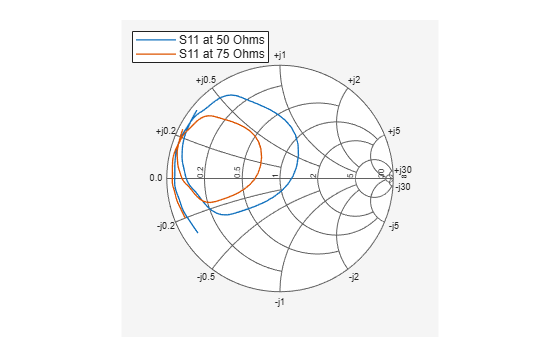## Syntax

``add(plot,data)``
``add(plot,frequency,data)``

## Description

example

````add(plot,data)` adds data to an existing Smith chart.```
````add(plot,frequency,data)` adds data to an existing Smith chart based on multiple data sets containing frequencies corresponding to columns of data matrix.```

## Examples

collapse all

```amp = read(rfckt.amplifier,'default.s2p'); Sa = sparameters(amp); figure smithplot(Sa,[1,1]) ```

Plot S-parameter object with new impedance of Z0 = 75 Ohms.

```Sa = sparameters(Sa,75); S11 = rfparam(Sa,1,1); Freq = Sa.Frequencies; s = smithplot('gco'); add(s, Freq, S11); s.LegendLabels = {'S11 at 50 Ohms', 'S11 at 75 Ohms'}; ```## Input Arguments

collapse all

Smith chart handle, specified as a function handle. If the handle of the Smith chart is not retained during creation, it is obtained by using the command `p = smithplot('gco')`.

Data Types: `double`

Input data, specified as a complex vector or complex matrix.

For a matrix D, the columns of D are independent data sets. For N-by-D arrays, dimensions 2 and greater are independent data sets.

Data Types: `double`
Complex Number Support: Yes

Frequency data, specified as a real vector.

Data Types: `double`

## Version History

Introduced in R2017b